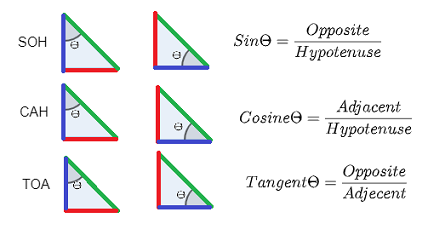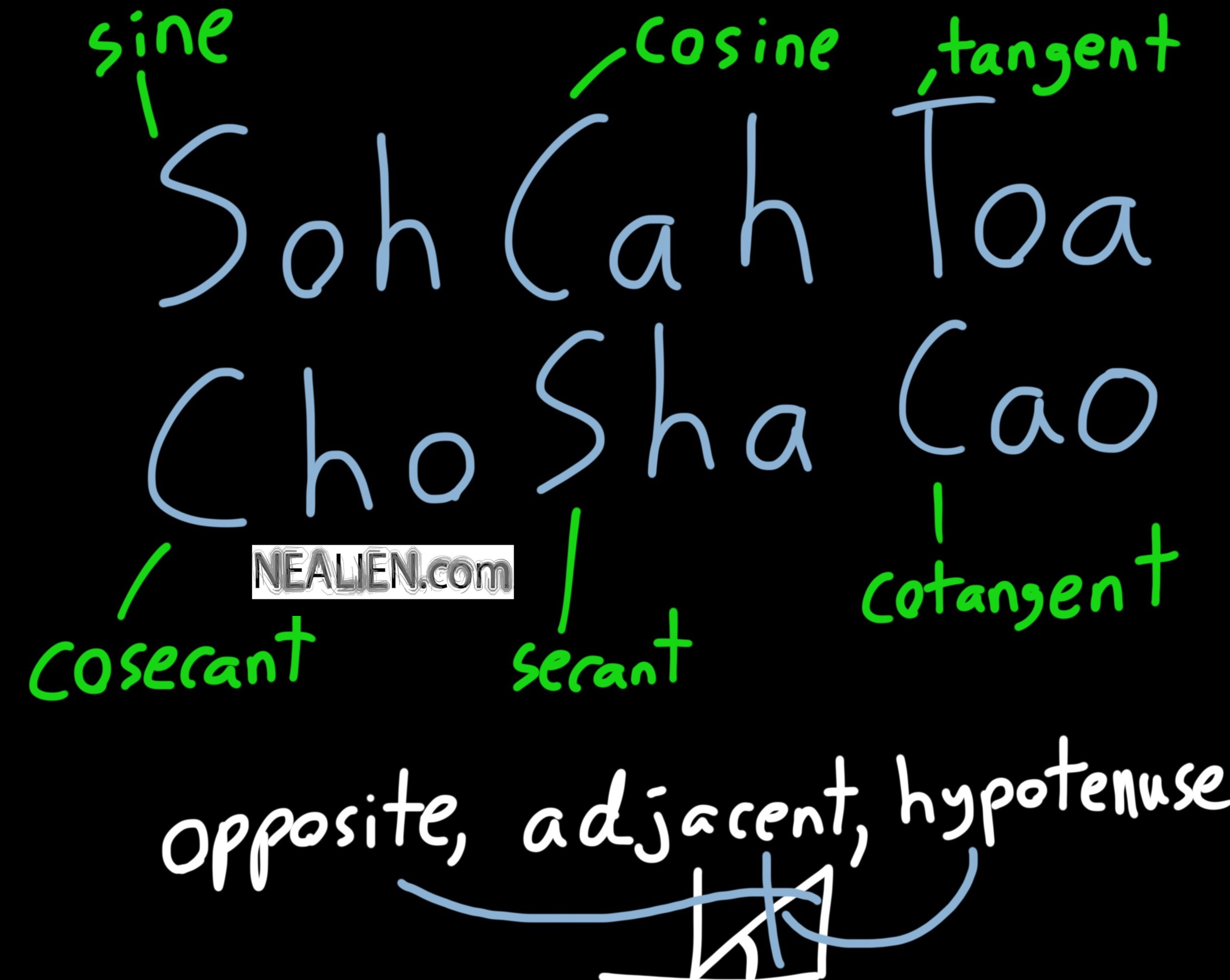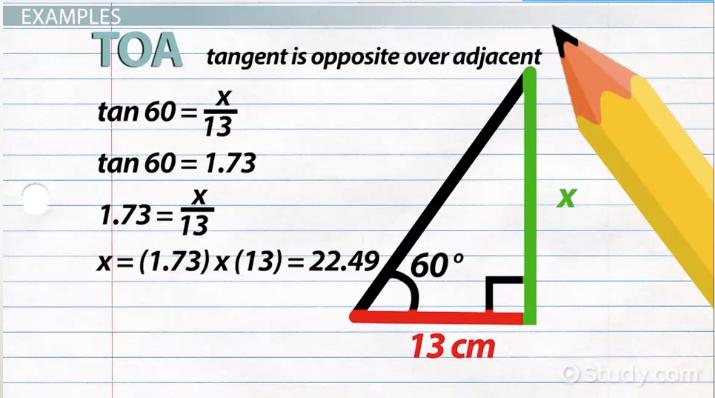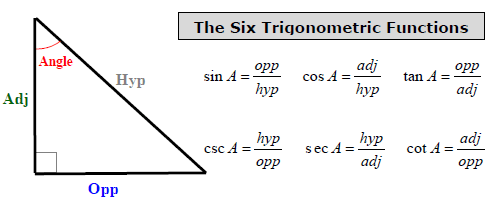# SOHCAHTOA: The easy way to remember trigonometric ratios

Trigonometry is one of the most basic topics of mathematics, but it is not easy. Trigonometry deals with angles, and triangles. The word itself comes from the Greek trigōnon (which means “triangle”) and metron (which means “measure”). It does the work of defining the relationship between the angles and sides of the triangles. The trigonometric ratios, their values for different angles, and many other aspects often become a nightmare for students. However, there is an innovative way for students to memorise the three most fundamental trigonometric ratios: SOHCAHTOA.

## What is sohcahtoa?The word sohcahtoa is a mnemonic. It helps to remember the basic trigonometric ratios, that is, sine (sin), cosine (cos), and tangent (tan). As we already know, trigonometric ratios are basically used in mathematical applications involving a triangle in any form. If we take a right-angled triangle, then the sine (sin) is the ratio of its height (length of the perpendicular drawn from the base) to the length of its hypotenuse. On the other hand, the cosine (cos) is the ratio of the length of the base of this triangle to the length of its hypotenuse. Finally, the tangent (tan) is the ratio of the triangle’s height to the length of the base of this triangle.

SOHCAHTOA stands for Sine, Opposite, Hypotenuse, Cosine, Adjacent, Hypotenuse, Tangent, Opposite, Hypotenuse. Now, let us see why it is framed so.

Sine (S) = Opposite (O) / Hypotenuse (H)

Cosine (C) = Adjacent (A) / Hypotenuse (H)

Tangent (T) = Opposite (O) / Adjacent (A).

The word Opposite in these formulae denotes the length of the side opposite, that is, the height, to the angle whose sine we are calculating. Similarly, since the base lies adjacent to the angle whose cosine we will calculate, the word adjacent is used here to represent the length of the base. Therefore, looking at the letters in parentheses next to the elements in the equations above, we see SOH, then CAH, and the TOA. Combining all gives us SOHCAHTOA. So, this is how we got this mnemonic to aid us in remembering trigonometric formulae.

## Sohcahtoa calculatorOver the internet, there are several websites which provide the service of calculating the sohcahtoa and other trigonometric functions of angles and sides of a triangle. One only needs to know the particulars about the triangle they are working on, like some variables. The most standard process is to first find out which two out of the hypotenuse, adjacent, opposite, and angle you have and then find the rest of the values. Then decide which relation you want to find out, fill the values in the calculator, and get the results.

Some calculators will simply have you put the values and select the ratio you want the value of. They will do the math and provide you with the result. However, these things can be done manually and far more handily on a calculator; you only need to know the values and the formulae which sohcahtoa helps immensely with.

Read Also: Ground State Electron Configuration

Smartphones these days also have a scientific calculator preinstalled on them. Just putting the values for the required results will solve the problem. Some standard values are advised to be memorised for their uniqueness and high chances of occurrence in questions, like tan 90° equals one (1), cosine 0° also equals 1. Sine for the angle 45 degrees is 1/√2, or roughly 0.7071.

## Sohcahtoa formulaSohcahtoa is a mnemonic. Each letter used in this word stands for something to do with the three basic trigonometric formulae.

Sin (θ) = Opposite / Hypotenuse

Cos (θ) = Adjacent / Hypotenuse

Tan (θ) = Opposite / Adjacent

Regardless of repetition, the three formulae above have in total nine words used (ignoring the theta (θ) in parentheses). Sohcahtoa also has nine letters in its spelling. In sohcahtoa, ‘s’ is for sin, ‘o’ for opposite, ‘h’ for the hypotenuse, ‘c’ for cos, ‘a’ for adjacent, and ‘t’ for tan. Reading this mnemonic in this manner will lay open before the reader the three formulae in a sequence and it will remain in their mind for a long time. Studying examples and solving problems by yourself will be the best kind of help if you want to make sure you never forget these three trigonometric formulae.

## Sohcahtoa trigonometryImagine you have studied very hard but there is a multitude of formulae you have to mug up. It often gets very tedious to remember so many formulae, especially in mathematics and related subjects, and those subjects which use mathematics a lot, like physics and statistics. In such dire times, shortcuts come as a welcome relief. Trigonometry is not alien to giving such feelings to its students. Trigonometry is one of the most important arms of mathematics and comes with its own set (certainly a big one) of formulae which are often difficult to remember in a conventional manner. This is where sohcahtoa comes to the rescue. Sohcahtoa is a very innovative way of memorising the three most primary trigonometric formulae, sine (sin), cosine (cos), and tangent (tan).

Sin (θ) = Opposite / Hypotenuse

Cos (θ) = Adjacent / Hypotenuse

Tan (θ) = Opposite / Adjacent

It is a mnemonic where ‘s’ is for sin, ‘o’ for opposite, ‘h’ for the hypotenuse, ‘c’ for cos, ‘a’ for adjacent, and ‘t’ for tan. Another thing, when we are finding out the ratios, there certainly is no other mathematical operator used apart from division.

So, when we read sohcahtoa in this sequence, first we get sine, opposite, hypotenuse. The student now only uses his common sense and divides the opposite by the hypotenuse, since it is a ratio, and gets the sine formula. Similarly, the next three we get are cos, adjacent, and hypotenuse. Again, the second word is divided by the third to get the value of the first word (cos, here). Lastly, for tangent (tan), follow the same method. The toa in sohcahtoa gives tan, opposite, and adjacent. Divide the opposite by the adjacent as per the formula and get the value of the tangent.

## Inverse sohcahtoaJust the way we can use sohcahtoa to remember the three fundamental trigonometric formulae, we can use inverse sohcahtoa to find the measure of an angle in a right-angled triangle. In finding these, sin-1, cos-1, and tan-1 will be helpful.

Commonly, we estimate the value of the third angle in a triangle by subtracting the other two angles from 180° (one hundred and eighty degrees) since the sum of all interior angles of a triangle is 180°. For example, if there is a right-angled triangle LMN, and we know two of its angles, say one is, of course, 90° (∠LMN), and the other is, say, 16° (∠NLM), then the third angle (∠LNM) would be

180° – (∠LMN) – (∠NLM) = (∠LNM)

=>  180° – 90° – 16° = 74°.

Alternatively, we can use the inverse sohcahtoa to find the same. But for that, we need to know the side lengths. Suppose the same triangle as assumed above with the same angles has the following dimensions:

LN (hypotenuse) = 76.19 cm, MN (adjacent) = 21 cm, and LM (opposite) = 73.24 cm. By virtue of sohcahtoa, we know sin (θ) = opposite / hypotenuse, where θ is any angle. Applying this to our formula, we can find angle LNM.

sin (∠LNM) = (73.24 / 76.19)

=> ∠LNM = sin-1 (73.24 / 76.19) = 74°.

## Sohcahtoa and choshacaoJust the way sohcahtoa is a way to remember the three elementary trigonometric formulae, choshacao is a way to remember the reciprocals of the very same formulae. The reciprocal of sine (sine) is cosecant (cosec), the reciprocal of cosine (cos) is secant (sec), and the reciprocal of tangent (tan) is cotangent (cot).

Since

Sine (S) = opposite (O) / hypotenuse (H),

=>  1 / sine = cosecant (C) = hypotenuse (H) / opposite (O)

Cosine (C) = adjacent (A) / hypotenuse (H)

=> 1 / cosine = secant (S) = hypotenuse (H) / adjacent (A)

and

Tangent (T) = opposite (O) / adjacent (A)

=> 1 / tangent = cotangent (C) = adjacent (A) / opposite (O).

Again, just like for sohcahtoa, if we take the first letter of the words cosecant, hypotenuse, opposite, secant, hypotenuse, adjacent, cotangent, adjacent, and opposite, in the same order, we get choshacao. This mnemonic is very similar to sohcahtoa in the sense that it is to remember the reciprocals of sine, cosine, and tangent. Cosecant, secant, and cotangent are also extremely important in the field of trigonometry, mathematics, and those subjects which involve the use of mathematics in their theory and applications.

## Sohcahtoa activityLearning anything is not enough unless we know when and how to apply them correctly, especially in mathematics which has arguably the widest range of applications, ranging from our day-to-day life to high-end and sophisticated calculations under advanced studies and research. Putting to use what we learn is extremely important, and practising that even more. The maxim “practice makes a man perfect” is enormously applicable in mathematics too.

Trigonometric activities involving sohcahtoa, and for that matter, choshacao, are available in school textbooks in a decent amount with varying levels of difficulty. Trigonometry is not taught in just one standard but in at least three to four standards in school. That provides an ample amount of practice questions to students. Then when one pursues higher education in technical fields and other subjects where mathematics is used extensively, like economics, they get a lot of opportunities to apply their trigonometric knowledge learnt earlier in order to polish themselves and get better at it. For even more enthusiastic students, the internet comes to the rescue. Questions involving sohcahtoa and choshacao are readily available for anyone who wants to practice and at all possible levels of difficulty. Following these will definitely lead students who want to learn trigonometry to expertise in the same.

## Sohcahtoa example problemsSohcahtoa is a mnemonic to remember the first three trigonometric formulae: sine, cosine, and tangent.

Let us work out some examples for sohcahtoa so you understand how to go about trigonometric problems.

Example 1:

Suppose a right-angled triangle has the following dimensions: one of the angles is 20° and the height (opposite) = 10 cm. Then, the hypotenuse of this triangle is

Sin 20° = opposite / hypotenuse

=> hypotenuse = opposite / sin 20°

=> hypotenuse = 10 / sin 20°

And therefore,

=> hypotenuse = 29.24 cm

Example 2:

Suppose a right-angled triangle has the following dimensions: opposite (height) = 10 cm and hypotenuse = 14 cm. The angle to which the opposite length (height) lies be θ. The value of θ is calculated as follows:

Sin θ = opposite / hypotenuse

=> sin θ = 10/14

=> θ = sin-1 (10/14)

Thus,

=> θ = 45.58°.

Example 3:

Suppose a right-angled triangle has the following dimensions: adjacent (base) = 8 cm and hypotenuse = 21 cm. The cosine of the aforementioned right-angled triangle would thus be

Cos = 8/21.

The angle whose cosine we just found be out would be θ. The value of θ will be

Cos θ = 8/21

=> θ = cos-1 (8/21)

=> θ = 67.61°.

## FAQs

### What is the formula for SOH CAH TOA?

SOHCAHTOA is a mnemonic which we use to remember the three primary trigonometric ratios, sine, cosine, and tangent. The formula for SOH CAH TOA, or more commonly, SOHCAHTOA, is

SOH: Sine (S) = Opposite (O) / Hypotenuse (H)

CAH: Cosine (C) = Adjacent (A) / Hypotenuse (H)

TOA: Tangent (T) = Opposite (O) / Adjacent (A).

### How do you know when to use sin Cos or tan?

It is very easy to know when to use sine, cosine, or tangent when you are working on a triangle. The formula for sine is opposite (height) divided by hypotenuse. So, when the question involves height and hypotenuse, we use sine. To find the cosine of an angle, we divide the adjacent (base) of the triangle by its hypotenuse. Thus, we can use cosine when we are posed with a problem that includes base and hypotenuse. Last but not the least, if the opposite and adjacent are being used, we use tangent as the formula for tangent is the ratio of the opposite to the adjacent.

### What is SOHCAHTOA exercise?

SOHCAHTOA exercises and activities are essentially practice materials for students of trigonometry. To practice any form of art is important to excel at it, and mathematics is not alien to it. The same goes for the branches of mathematics. SOHCAHTOA exercises are available in abundance in mathematics textbooks and over the internet.

### How do you remember 6 trig functions?The three most basic trigonometric functions, sine, cosine, and tangent, along with their reciprocals, cosecant, secant, and cotangent, form the base of trigonometry. Needless to say, it is important to know them, remembering the relations can often be difficult. However, there are effective ways available to remember them. SOHCAHTOA is a mnemonic made to remember sine, cosine, and tangent. Similar to it is CHOSHACAO, another mnemonic used to remember their reciprocals, cosecant, secant, and cotangent respectively.